Understanding Frequency Performance Specifications

Publish Date: Oct 14, 2019 | 10 Ratings | 3.60 out of 5 | Print

Overview

Electrical test instruments come with extensive specification manuals and data sheets that provide measurement performance data. Those documents include timing and triggering constraints, DC and AC accuracy, and frequency domain performance. The most common specifications to judge the spectral quality or frequency domain performance of oscilloscopes and arbitrary waveform generators are flatness, spurious free dynamic range (SFDR), signal-to-noise ratio (SNR), total harmonic distortion (THD), signal-to-noise-and-distortion (SINAD) ratio, and effective number of bits (ENOB). Although all of these performance specifications can be useful, SINAD and ENOB are the most comprehensive. Read on to learn more.

1. Important Terms

Fourier Transform—A Fourier transform is a graphical representation of all frequency components that appear in a signal over a given period of time. The x-axis of a Fourier transform is in units of frequency. The y-axis of a Fourier transform shows the amplitude of the signal at a given frequency.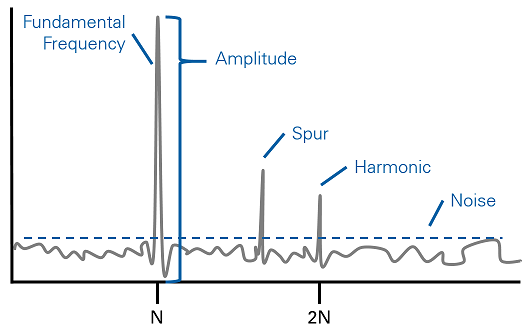Figure 1. Many of the important terms to understand with frequency performance can be visualized together on a Fourier transform.

Amplitude—Amplitude refers to the voltage or current level of signals at a given frequency. In a Fourier transform, the amplitude refers to the height of the histogram in that particular frequency bin. Power can also be used to describe the level of a given frequency or a integration of amplitudes over a given frequency range.

Fundamental Frequency—Also known as the carrier frequency, the fundamental frequency is the lowest frequency component of a periodic signal. In an ideal Fourier transform of a sine wave, only the fundamental frequency would appear. Most of the specifications below are generated using an almost ideal sine wave.

Harmonics – Harmonics, or harmonic frequencies, are frequencies that are integer multiples of the fundamental frequency. Harmonics are often not part of the actual signal, but appear due to Nyquist sampling theory and transmission line reflections. An ideal square wave will appear as a set of all harmonics of the fundamental frequency on a Fourier transform.

Spurs—Spurs, also known as device spurs, are frequency components that appear in signals because of the electrical components of the instrument. Some examples of spurs are interleaving anomalies in analog-to-digital converters (ADCs), leakage of oscillator clock signals, and power inserted by amplifiers.

Noise—All voltage and frequency components that are not present in the actual or ideal signal, spurs, or harmonics, but are present in the measurement or generation of test signals, are noise. Some common causes of noise are environmental magnetic fields, temperature, and ground loops. The noise floor is the maximum Fourier transform amplitude of any noise in the device’s frequency range.

Front End—The front end of an instrument is all components external to the converter including analog amplifiers and filters.

2. Flatness

Flatness, or passband flatness, specifies the limits within which the amplitude of a signal varies throughout a device’s operating frequency range and is usually expressed in decibels (dB). Flatness is important when measuring signals that have a wide range of frequency components. As the frequency of any incoming waveform changes, the impedance of the device front end varies. Impedance is the sum of resistance and capacitance of a transmission path and changes with frequency. The change in impedance affects the amplitude of signals, particularly higher bandwidth signals near the -3 dB roll-off point of the instrument’s bandwidth. Figure 1 shows two signals: one is measured with an oscilloscope that has very good passband flatness and the other is measured with an oscilloscope that has poor passband flatness.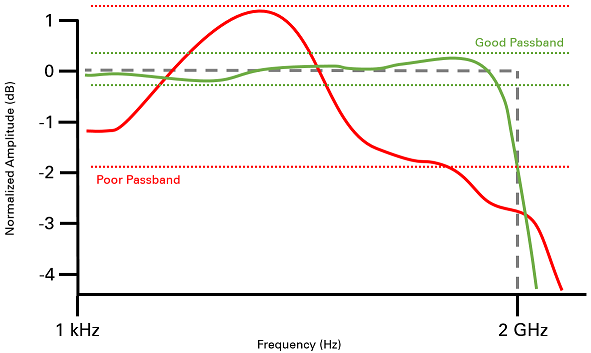Figure 2. An instrument with good passband flatness (represented in green) would generate or measure a signal with very consistent amplitude throughout the operating bandwidth. Some instruments have poor passband flatness performance (represented in red) and have to be corrected.

Flatness, then, really describes how well the analog front end maintains the amplitude of incoming signals of different frequencies to the ADC of an oscilloscope or the outgoing signals from the digital-to-analog converter (DAC) of an arbitrary waveform generator. Digital filtering and correction are implemented in many instruments to account for non-flatness over frequency. A maximally flat front end passes all frequencies with the same amount of attenuation, which results in an accurate signal that can be easily correlated.

If an instrument has very good flatness performance, you can trust it to be accurate no matter the frequency of the signal of interest. Great flatness performance means no need for engineers to do correction on the instrument circuitry or data, and simple correlation of test results.

3. Spurious Free Dynamic Range

SFDR is the usable dynamic range before spurious noise interferes with or distorts the fundamental signal. The equation used to calculate SFDR is the measure of the ratio in amplitude between the fundamental signal and the largest related spur from DC to the full Nyquist bandwidth. The difference between the amplitude of the fundamental frequency and the amplitude of the maximum spur is the dynamic range. SFDR is expressed in dBc (with respect to the carrier frequency amplitude) or dBFS (with respect to the full scale of the ADC). Figure 2 shows a Fourier transform depicting the carrier frequency, or fundamental frequency, of the signal as well as the spur with the most energy that defines the SFDR.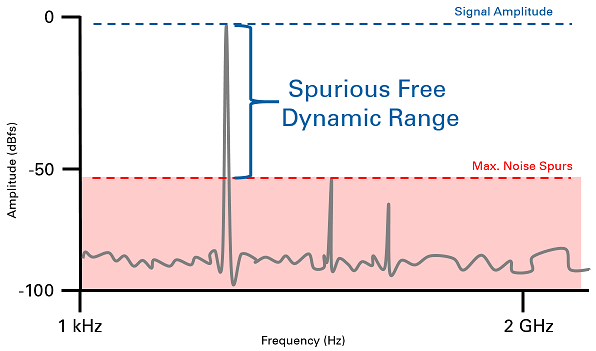Figure 3. SFDR is a ratio of the signal fundamental frequency amplitude to the maximum amplitude of spurs or noise. Instruments with high SFDR can measure or generate a signal with high fidelity.

An instrument that has very low noise and device spurs is said to have very high dynamic range or a very low noise floor. This means that a device with high SFDR can measure or generate a signal with very few effects from noise and spurs.

4. Signal-to-Noise Ratio

SNR is the ratio of the power of the input signal level to the power of the noise level and is usually expressed in dB. It can also be calculated using the root mean square (RMS) value of the signal amplitude and the noise amplitude as shown in Equation 1.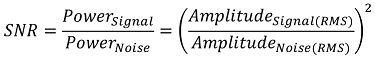Equation 1. SNR is the ratio of signal to noise power. The best approximation of SNR is using power of the amplitude and noise, but the most accurate calculation uses the RMS quantization of each.

When calculating SNR, the bandwidth of the signal should be specified. The larger the SNR specification, the better an instrument differentiates the signal from the noise in measurements and generations. Better definition of the signal of interest means better frequency and AC voltage or current performance and resolution of signals with low energy. Figure 3 shows a representation of SNR as it appears on a Fourier transform.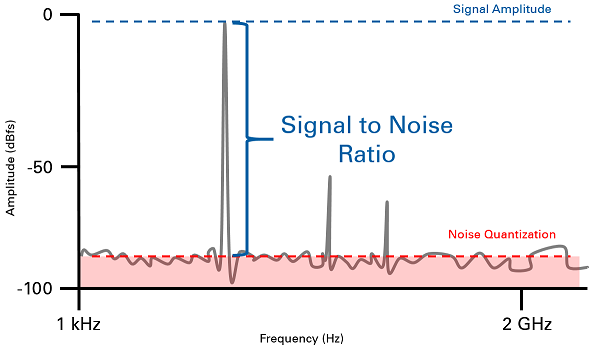Figure 4. SNR shows how well signal frequency components will stand out from noise in a device’s measurements and generations, but does not account for the effects of device spurs.

5. Total Harmonic Distortion

The THD of a signal is the ratio of the sum of the powers of the first five harmonics to the power of the fundamental frequency. Equation 2 shows the calculation for THD where H is the amplitude of each harmonic and F is the amplitude of the fundamental frequency.

.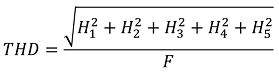Equation 2. THD is calculated by dividing the power of the first five harmonics of a signal by the power of the fundamental frequency that appear in a measurement or generation.

THD can be expressed in dB, dBc, or percent. Measurements for calculating the THD are made at the output of a device under specified conditions.

THD expresses the effect of high amplitude frequency components that are included in measurements or generations of a given signal frequency on the instrument. A device with high THD at a given frequency will be less accurate because of the effect of harmonics than at other frequencies or other instruments with lower THD.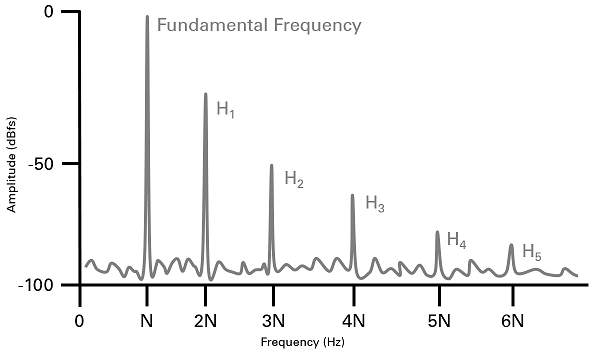Figure 5. THD is particularly useful for lower frequency signals in which all of the five harmonics are included in the instruments operating frequency range. This figure shows what it looks like when all these THD frequencies appear in the device passband.

6. Signal-to-Noise-and-Distortion

SINAD, also known as THD plus noise (THD+N), is the ratio of the RMS signal amplitude (set to 1 dB below full scale) to the RMS sum of all other spectral components. All other spectral components include the harmonics but exclude DC. The most useful approximation of SINAD is the power of the fundamental signal frequency, plus the power of device spurs and power of noise divided by the power of noise plus the power of distortion, as shown in Equation 3.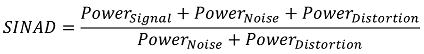Equation 3. The calculation of SINAD includes the power of frequency components in the signal of interest, noise, and distortion.

SINAD is usually expressed in dB. Figure 6 shows SINAD as represented in a Fourier transform.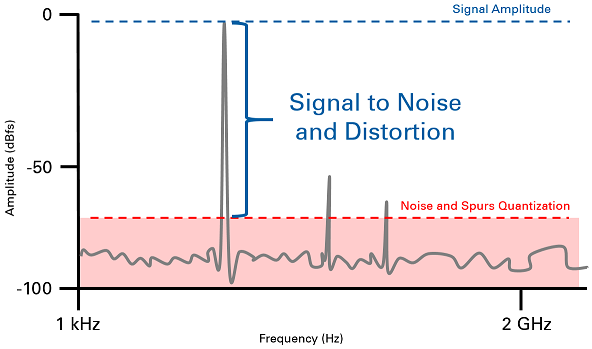Figure 6. SINAD shows how well signal frequency components will stand out from noise and spurs in a device’s measurements and generations. Devices with large SINAD have more accurate frequency and amplitude accuracy.

Although all of the specifications listed above can be useful, SINAD is the single most useful measure of instrument performance because it accounts for all power of noise and spurs in the instrument’s operating frequency range. An instrument with high SINAD can better differentiate the frequency components in the signal of interest from spurs and noise. Better definition of the signal means more accurate frequency and AC voltage or current performance and resolution of signals with low energy.

7. Effective Number of Bits

ENOB is a specification that relates measurement or generation performance of a device to a common specification used in data converters: bits of resolution. Most data converters are designed to perform at a particular speed and resolution. Instrument vendors have always used this design element to define the measurement resolution of their devices. As mentioned previously, no instrument is ideal, so performance specifications show how close a device is to ideal. ENOB is calculated directly from SINAD using values for ideal ADC noise and spurs as shown in Equation 4. This calculation shows how close to an ideal instrument the device is performing.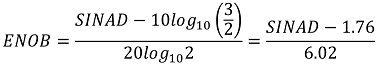Equation 4. ENOB is calculated directly from SINAD and is a specification that relates the instrument's actual resolution performance to the resolution specification on the front panel.

A device that has an ENOB specification very close to the resolution specification of the data converter used in its design is said to be well designed. For instance, an oscilloscope that uses a 14-bit ADC and has ENOB of 11.5 bits is a good instrument because it has effectively 2,896 bins to measure voltage levels (as opposed to 16,384). An 8-bit oscilloscope with ENOB of 4 is not a good instrument because it has effectively 16 bins to measure values (as opposed to 256).

Ratings

Rate this document